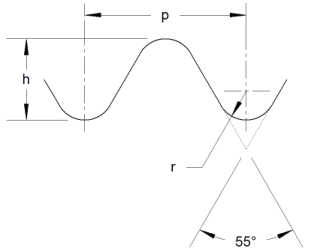Search:

# Model Engineer (M.E.)p = Pitch = 1/TPI h = Basic Depth of Thread = 0.640327p r = Basic Radius = 0.137329p